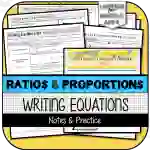Math > Algebra | Grade 7 | Activities

# Writing Equations for Proportional Relationships NOTES & PRACTICEAttributes
Subject

Algebra

Types

Activities

File

PDF

Editable
No
RatingThis resource was developed to meet the requirements of the 7th Grade Ratios & Proportional Relationships Standards below:

CCSS.MATH.CONTENT.7.RP.A.2 Recognize and represent proportional relationships between quantities.

CCSS.MATH.CONTENT.7.RP.A.2.B Identify the constant of proportionality (unit rate) in tables, graphs, equations, diagrams, and verbal descriptions of proportional relationships.

CCSS.MATH.CONTENT.7.RP.A.2.C Represent proportional relationships by equations. For example, if total cost t is proportional to the number n of items purchased at a constant price p, the relationship between the total cost and the number of items can be expressed as t = pn.

## What's Included

This resource contains the following items:

1) Writing Equations for Proportional Relationships NOTES & PRACTICE: Students will use their prior knowledge of identifying the Constant of Proportionality in order to write equations for the following proportional relationship formats:

· Tables

· Diagrams

· Graphs

· Real-World Problems (Descriptions, Tables, & Graphs)

2) Writing Equations for Proportional Relationships TEST PRACTICE (Printable and version for Google Forms)

3) Answer Key to All Parts

## Resource Tags

proportional relationships writing equations notes

## 0 Reviews

### you may also like...

Check out these other great products